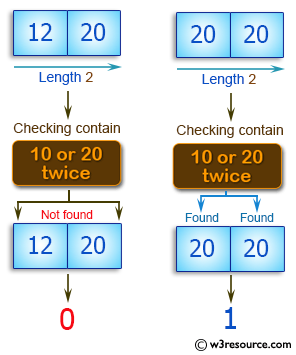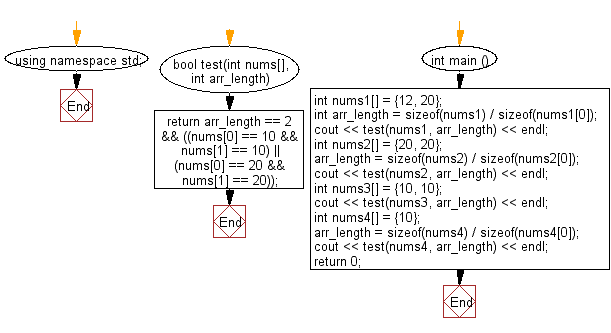﻿ C++ : Check if an array of integers contains 10 or 20 twice# C++ Exercises: Check a given array of integers and return true if the array contains 10 or 20 twice

## C++ Basic Algorithm: Exercise-91 with Solution

Write a C++ program to check a given array of integers and return true if the array contains 10 or 20 twice. The length of the array will be 0, 1, or 2.

Sample Solution:

C++ Code :

``````#include <iostream>
using namespace std;

bool test(int nums[], int arr_length)
{
return arr_length == 2
&& ((nums == 10 && nums == 10)
|| (nums == 20 && nums == 20));
}

int main () {
int nums1[] = {12, 20};
int arr_length = sizeof(nums1) / sizeof(nums1);
cout << test(nums1, arr_length) << endl;
int nums2[] = {20, 20};
arr_length = sizeof(nums2) / sizeof(nums2);
cout << test(nums2, arr_length) << endl;
int nums3[] = {10, 10};
cout << test(nums3, arr_length) << endl;
int nums4[] = {10};
arr_length = sizeof(nums4) / sizeof(nums4);
cout << test(nums4, arr_length) << endl;
return 0;

}
``````

Sample Output:

```0
1
1
0
```

Pictorial Presentation:Flowchart:C++ Code Editor:

Contribute your code and comments through Disqus.

What is the difficulty level of this exercise?

﻿